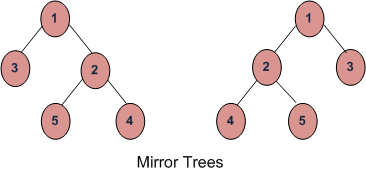# Check if two trees are Mirror | Set 2

Given two Binary Trees, returns true if two trees are mirror of each other, else false.
Mirror Tree :Previously discussed approach is here.

## Recommended: Please try your approach on {IDE} first, before moving on to the solution.

Approach :
Find the inorder traversal of both the Binary Trees, and check whether one traversal is reverse of another or not. If they are reverse of each other then the trees are mirror of each other, else not.

## CPP

 `// CPP code to check two binary trees are ` `// mirror. ` `#include ` `using` `namespace` `std; ` ` `  `struct` `Node ` `{ ` `    ``int` `data; ` `    ``Node* left, *right; ` `}; ` ` `  `// inorder traversal of Binary Tree ` `void` `inorder(Node *n, vector<``int``> &v) ` `{ ` `    ``if` `(n->left != NULL) ` `    ``inorder(n->left, v);         ` `    ``v.push_back(n->data);     ` `    ``if` `(n->right != NULL) ` `    ``inorder(n->right, v);  ` `} ` ` `  `// Checking if binary tree is mirror  ` `// of each other or not. ` `bool` `areMirror(Node* a, Node* b) ` `{ ` `  ``if` `(a == NULL && b == NULL) ` `    ``return` `true``;     ` `  ``if` `(a == NULL || b== NULL) ` `    ``return` `false``; ` `  `  `  ``// Storing inorder traversals of both  ` `  ``// the trees. ` `  ``vector<``int``> v1, v2; ` `  ``inorder(a, v1); ` `  ``inorder(b, v2); ` ` `  `  ``if` `(v1.size() != v2.size()) ` `     ``return` `false``; ` ` `  `  ``// Comparing the two arrays, if they  ` `  ``// are reverse then return 1, else 0 ` `  ``for` `(``int` `i=0, j=v2.size()-1; j >= 0; ` `                             ``i++, j--) ` `     `  `      ``if` `(v1[i] != v2[j]) ` `        ``return` `false``;     ` `     `  `  ``return` `true``; ` `} ` ` `  `// Helper function to allocate a new node ` `Node* newNode(``int` `data) ` `{ ` `  ``Node* node = ``new` `Node; ` `  ``node->data  = data; ` `  ``node->left  =  node->right  = NULL; ` `   `  `  ``return``(node); ` `} ` `  `  `// Driver code ` `int` `main() ` `{ ` `  ``Node *a = newNode(1); ` `  ``Node *b = newNode(1); ` `   `  `  ``a -> left = newNode(2); ` `  ``a -> right = newNode(3); ` `  ``a -> left -> left  = newNode(4); ` `  ``a -> left -> right = newNode(5); ` `  `  `  ``b -> left = newNode(3); ` `  ``b -> right = newNode(2); ` `  ``b -> right -> left = newNode(5); ` `  ``b -> right -> right = newNode(4); ` `  `  `  ``areMirror(a, b)? cout << ``"Yes"` `: cout << ``"No"``; ` `  `  `  ``return` `0; ` `} `

Output:

```Yes
```

This article is contributed by Anuj Chauhan. If you like GeeksforGeeks and would like to contribute, you can also write an article using contribute.geeksforgeeks.org or mail your article to contribute@geeksforgeeks.org. See your article appearing on the GeeksforGeeks main page and help other Geeks.

My Personal Notes arrow_drop_upCheck out this Author's contributed articles.

If you like GeeksforGeeks and would like to contribute, you can also write an article using contribute.geeksforgeeks.org or mail your article to contribute@geeksforgeeks.org. See your article appearing on the GeeksforGeeks main page and help other Geeks.

Please Improve this article if you find anything incorrect by clicking on the "Improve Article" button below.

Article Tags :
Practice Tags :

Be the First to upvote.

Please write to us at contribute@geeksforgeeks.org to report any issue with the above content.